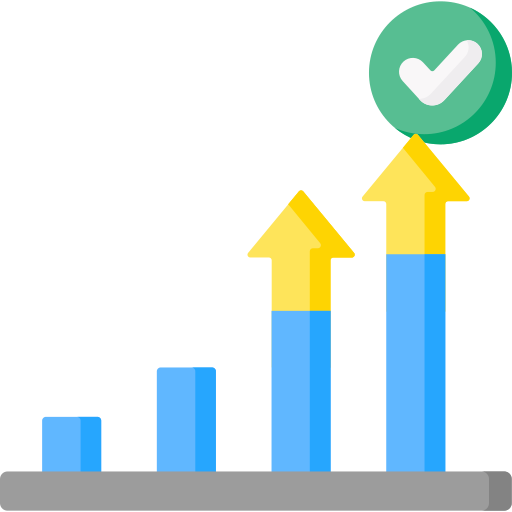# Pie Chart Questions and Answers

## Pie Charts Questions and Answers

Go through the entire page to know about Pie charts and also practice Pie Chart Questions and Answers at the end.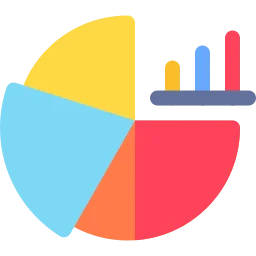## What is a Pie Chart ?

A pie chart is a circularly shaped geometrical visual graphic that exhibits data, information, figures, and statistics in the format of an easy-to-read ‘pie slice’ with fluctuating sizes describing how much one data component exists. The larger the part, the more of that precise data were grouped.

### How to solve Pie Chart Questions and Answers

• Start with what you want to illustrate: The main topic will be the title of your chart.
• Gather the data: Pie charts depict percentages. Make sure your percentages add up to 100% and get rid of any rounding issues that would make the total something other than 100%. SmartDraw allows you to write the percentage directly into a pie chart template or you can drag the edge of a wedge to make it bigger or smaller.
• Consider creating a legend: If a wedge is too small to hold text, you can create a label and draw a line identifying the appropriate slice. Alternatively, you can create a key that shows what each color represents.

### Some Important Points about Pie Chart:

• Pie charts are easy to understand and require minimal effort to interpret.
• Pie charts are ideal for displaying data that represents parts of a whole, such as budget allocations, market share, or the composition of a population.
• They are visually appealing and can make your data presentation more engaging and memorable.

### Below is a Sample image to understand what Pie chart is

The below image shows the expenditure of Mukesh and Nita in terms of a Pie chart.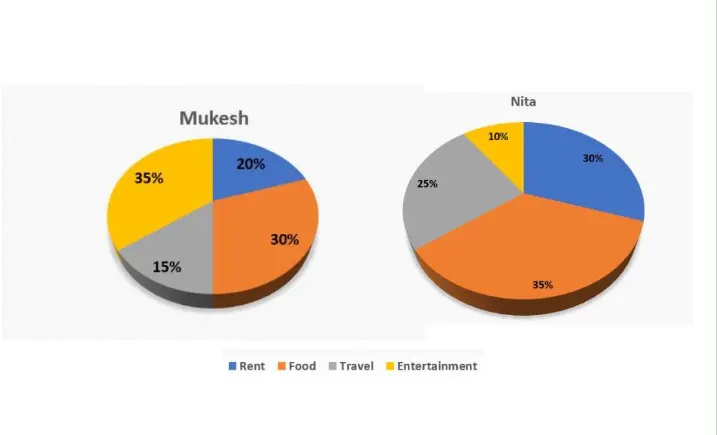### Related Banners

Get PrepInsta Prime & get Access to all 200+ courses offered by PrepInsta in One Subscription

## Pie charts Questions and Answers

1. The following pie-chart displays the percentage distribution of the cost in self-publishing a book. Study the pie-chart below and then answer the questions accordingly.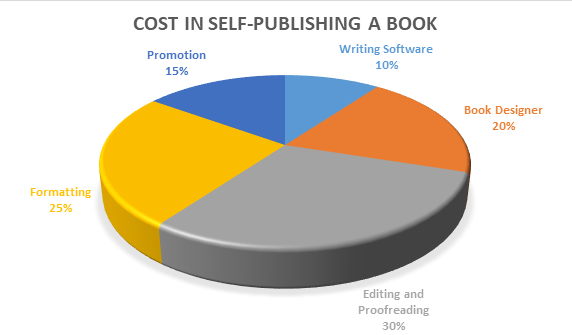If for certain number of books, the publisher paid the amount of Rs. 21,500 for the cost for book designer, then how much amount will be paid for promotion of the book?16125

16125

72.16%

18559

18559

11.34%

12489

12489

8.25%

16000

16000

8.25%

Let the amount paid for promotion be x
Then, 20: 15 = 21500: x
x = 21500 * 15/20 = 16125
Therefore, the amount paid for promotion is Rs. 161252. The following pie-chart displays the percentage distribution of the cost in self-publishing a book. Study the pie-chart below and then answer the questions accordingly.Find the sector’s central angle which is corresponding to the amount paid on Book Designing?$54^{o}$

$54^{o}$

21.95%

$68^{o}$

$68^{o}$

18.29%

$72^{o}$

$72^{o}$

54.88%

$81^{o}$

$81^{o}$

4.88%

Sector’s central angle corresponding to Book Designing: 20% of 360o

= 20/100 * 360 = 72o.3. The following pie-chart displays the percentage distribution of the cost in self-publishing a book. Study the pie-chart below and then answer the questions accordingly.If 4200 copies of the book are published and its cost for editing and proofreading cost is 65800, then find at what price the seller will sell the book if he wants to earn 20% profit?61.5

61.5

11.48%

62.66

62.66

59.02%

69.05

69.05

9.84%

None of the above

None of the above

19.67%

For the seller wants to earn 20 % profit, then selling price = 120% of cost price.

Also editing and proofreading cost = 30% of C.P.

Then, let us assume the selling price of 4200 books as x

Then, 30: 120 = 65800: x

x = 120 * 65800 / 30 = 263200

Therefore, selling price of one book = 263200/4200 = Rs. 62.664. The following pie-chart displays the percentage distribution of the cost in self-publishing a book. Study the pie-chart below and then answer the questions accordingly.By how much amount the formatting of the book is a lesser amount for editing the book?25%

25%

14.04%

16.66%

16.66%

71.93%

51%

51%

7.02%

32%

32%

7.02%

: Editing Cost = 30% of the cost price of the book

Formatting cost = 25% of the cost price of the book

Difference = (30%) - (25%) = 5%

Therefore, percentage difference = 5% / Editing cost * 100

= 5/30 * 100 = 16.66%5. The pie-charts below displays the percentage distribution of people going out of India and the age group of those people. Study the pie-chart below carefully and then answer the questions accordingly.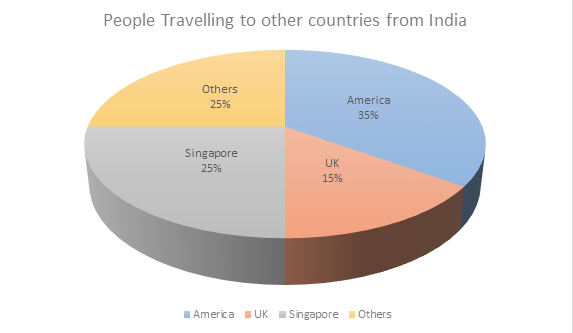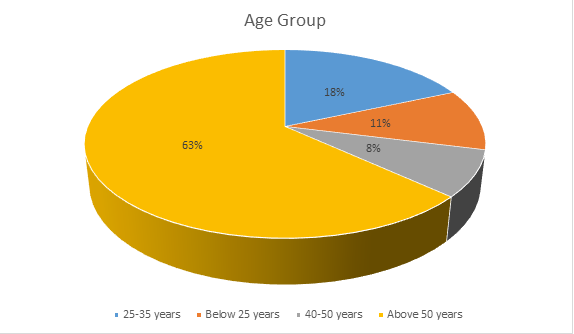What percent of Indian people went to UK or Singapore?35%

35%

14.55%

40%

40%

78.18%

41%

41%

5.45%

58%

58%

1.82%

According to the 1st chart above the Indian people went to UK is 15% and Indian people went to Singapore is 25%

Therefore, Indian people went to UK or Singapore = 15 + 25 = 40%.6. The pie-charts below displays the percentage distribution of people going out of India and the age group of those people. Study the pie-chart below carefully and then answer the questions accordingly.Find the ratio of the number of Indian people that went to America to the number of Indian people whose age was below 25 years.35:11

35:11

85.71%

22:7

22:7

5.36%

5:1

5:1

7.14%

10:3

10:3

1.79%

The percent of the number of Indian people that went to America = 35%

The percent of the number of Indian people whose age was below 25 years = 11%

Therefore, ratio = 35: 117. The pie-charts below displays the percentage distribution of people going out of India and the age group of those people. Study the pie-chart below carefully and then answer the questions accordingly.If between other countries, Germany accounted for 27% of the Indian people traveling, and it is recognized from records that a total of 20 lakh Indian tourists had gone to Germany during the year, then how many people of 25-35 year who went abroad in that year?18 lakh

18 lakh

37.5%

180 Lakh

180 Lakh

34.38%

175 Lakh

175 Lakh

9.38%

75 Lakh

75 Lakh

18.75%

Tourist from other countries to Germany is 25%

Among that 25%, 27% is Indian people

Therefore, 27% of 25% = 2% corresponding to Indian people in Germany

2% corresponds of Germany’s 20 Lakh.

1% = 10 lakh.

Therefore 18%(25-35 year of age) = 18 * 10 = 180 Lakh people.8. The following pie-charts displays the percentage distribution Indian market share by Value of different smartphone companies for the year 2009.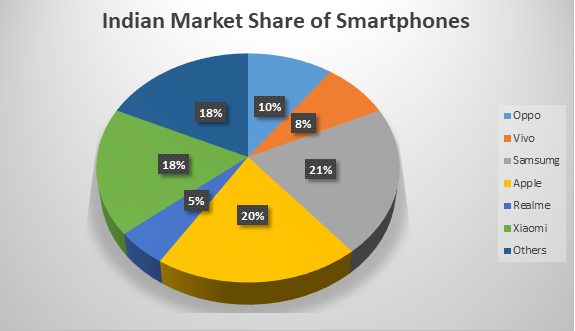The following pie-charts displays the percentage distribution Indian market share by Volume of different smartphone companies for the year 2009.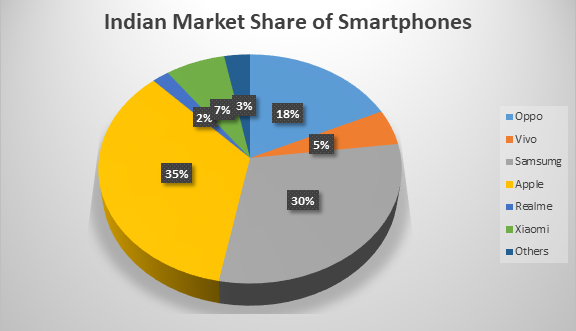Number of smartphones sold in 2009 in India = 2,500

Value of smartphones sold in 2009 in India = Rupees 45, 000,000

For the year 2009, what is the average unit sales price of Vivo smartphones?25500

25500

18.75%

27700

27700

15.63%

29500

29500

12.5%

28800

28800

53.13%

Vivo has 8% value share and 5% volume share.

8% of 45, 000,000 = 8 * 45000000/100 = 3600000

5% of 2500 = 5 * 2500/100 = 125

Therefore, average unit sale price = 3600000/125 = 288009. The following pie-charts displays the percentage distribution Indian market share by Value of different smartphone companies for the year 2009.The following pie-charts displays the percentage distribution Indian market share by Volume of different smartphone companies for the year 2009.Number of smartphones sold in 2009 in India = 2,500

Value of smartphones sold in 2009 in India = Rupees 45, 000,000

For the year 2009, what is the average unit sales price of Apple and Samsung smartphones?22886

22886

38.71%

22550

22550

19.35%

22850

22850

16.13%

22880

22880

25.81%

Apple has 20% value share and 35% volume share.

20% of 45, 000,000 = 20 * 45000000/100 = 9000000

35% of 2500 = 35 * 2500/100 = 875

Therefore, average unit sale price of Apple = 9000000/875 = 10286(Approximately)

Samsung has 21% value share and 30% volume share.

21% of 45, 000,000 = 21 * 45000000/100 = 9450000

30% of 2500 = 30 * 2500/100 = 750

Therefore, average unit sale price of Apple = 9450000/750= 12600

Therefore, the average unit sales price of Apple and Samsung smartphones = 10286 + 12600 = 2288610. The following pie-charts displays the percentage distribution Indian market share by Value of different smartphone companies for the year 2009.The following pie-charts displays the percentage distribution Indian market share by Volume of different smartphone companies for the year 2009.Number of smartphones sold in 2009 in India = 2,500

Value of smartphones sold in 2009 in India = Rupees 45, 000,000

For the year 2009, what is the average unit sales price of Apple and Samsung smartphones?22886

22886

72.41%

22550

22550

10.34%

22850

22850

10.34%

22880

22880

6.9%

Apple has 20% value share and 35% volume share.

20% of 45, 000,000 = 20 * 45000000/100 = 9000000

35% of 2500 = 35 * 2500/100 = 875

Therefore, average unit sale price of Apple = 9000000/875 = 10286(Approximately)

Samsung has 21% value share and 30% volume share.

21% of 45, 000,000 = 21 * 45000000/100 = 9450000

30% of 2500 = 30 * 2500/100 = 750

Therefore, average unit sale price of Apple = 9450000/750= 12600

Therefore, the average unit sales price of Apple and Samsung smartphones = 10286 + 12600 = 22886×